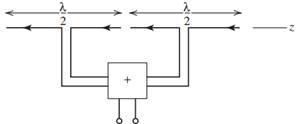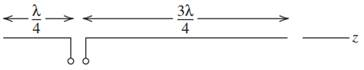### Create an Account

Home / Questions / The outputs from two collinear closely spaced half wave dipoles are added together as indi...

# The outputs from two collinear closely spaced half wave dipoles are added together as indicated by a summing device in the figure below The transmission lines from the antennas to the summer are of

The outputs from two collinear, closely spaced, half-wave dipoles are added together as indicated by a summing device in the figure below. The transmission lines from the antennas to the summer are of equal length. Write the pattern Fa(θ) of this antenna system using array techniques.(b) Now consider a center-fed, full-wave dipole that is along the z-axis. Write its pattern expression Fb(θ).

(c) Now draw the current distributions Ia(z) and Ib(z) for both antennas. From these current distributions, can you make a statement about the patterns from the two antennas? Return to the pattern expressions and prove your statement mathematically.Jun 22 2020 View more View LessSubscribe To Get Solution